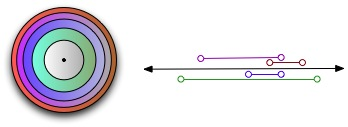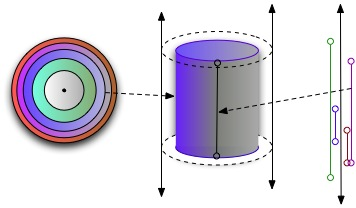# Multiplying Spaces

When people talk informally about topology, we always say that the basic idea of equivalence is that two spaces are equivalent if they can be bent, stretched, smushed, or twisted into each other, without tearing or gluing. A mug is the same shape as a donut, because you can make a donut out of clay, and then shape that donut into a mug without tearing, punching holes, or gluing pieces together. A sphere is the same shape as a cube, because if you’ve got a clay sphere, you can easily reshape it into a cube, and vice-versa.

Homeomorphism is the actual formal definition of that sense of equivalence. The intuition is fantastic – it’s one of the best informal description of a difficult formal concept that I know of in math! But it’s not ideal. WHen you take a formal idea and make it informal, you always lose some details.

What we’re going to do here is try to work our way gradually through the idea of transformability and topological equivalence, so that we can really understand it. Before we can do that, we need to be able to talk about what a continuous transformation is. To talk about continuous transformations, we need to be able to talk about some topological ideas called homotopy and isotopy. And to be able to define those, we need to be able to use topological products. (Whew! Nothing is ever easy, is it?) So today’s post is really about topological products!

The easiest way that I can think of to explain the product of two topological spaces is to say that it’s a way of combining the structures of the spaces by adding dimensions. For example, if you start with two spaces each of which is a line segment, the product of those two spaces is a square (or a circle, or an octagon, or …) You started with two one-dimensional spaces, and used them to create a new two-dimensional space. If you start with a circle and a line, the product is a cylinder.

In more formal terms, topological products are a direct extension of cartesian set products. As the mantra goes, topological spaces are just sets with structure, which means that the cartesian product of two topological sets is just the cartesian products of their point-sets, plus a combined structure that preserves attributes of the original structure of the spaces.

Let’s start with a reminder of what the cartesian product of two sets is. Given a set$A$ and a set$B$, the cartestian product$A \times B$ is defined as the set of all possible pairs$(a, b)$, where$a \in A$ and$b \in B$. If$A=\{1, 2, 3\}$ and$B=\{4, 5\}$, then$A\times B = \{ (1, 4), (1, 5), (2, 4), (2, 5), (3, 4), (3, 5) \}$.

In category theory, we take the basic idea of the cartesian product, and extend it to a general product of different mathematical objects. It does this by using the idea of projections. In this model, instead of saying that the product of sets$A$ and$B$ is a set of pairs$(a, b)$, we can instead say that the product is a set$S$ of objects, and two functions$P_A : S \rightarrow A$ and$P_B : S \rightarrow B$. (To be complete, we’d need to add some conditions, but the idea should be clear from this much.) Given any object in the the product set$S$,$P_A(S)$ will give us the projection of that object onto$A$. This becomes more interesting when we consider sets of objects. The A-projection of a collection of points from the product set$S$ is the shadow that those points cast onto the set A.

A topological product is easiest to understand with that categorical approach. The set of points in a product category$A \times B$ is the cartesian product of the sets of points in$A$ and the sets of points in$B$. The trick, with topologies, is that you need to describe the topological structure of the product set: you need to be able to say what the neighorhoods are. There are lots of ways that you could define the neighborhoods of the product, but we define it as the topological space with the smallest collection of open-sets. To understand how we get that, the projections of the category theoretical approach make it much easier.

Informally, the neighborhoods in the product$A \times B$ are things that cast shadows into the topological spaces$A$ and$B$ which are neighborhoods in$A$ and$B$.

Suppose we have topological spaces A and B. If$S$ is the product topology$A \times B$, then it has projection functions$P_A: S \rightarrow A$ and$P_B: S \rightarrow P_B$.

The projection functions from the product need to maintain the topological structure of the original topologies. That means that the projection function must be continuous. And that, in turn, means that the inverse image of the projection function is an open set. So: for each open set$O$ in$A$,$P_A^{-1}(O)$ is an open-set in$S$.

Let’s look at an example. We’ll start with two simple topological spaces – a cartesian plane (2d), and a line (1d). In the plane, the neighborhoods are open circles; in the line, the neighborhoods are open intervals. I’ve illustrated those below.The product of those two is a three dimensional space. The neighborhoods in this space are cylinders. If you use the projection from the product to the plane, you get open circles – the neighborhood structure of the plane. If you use the projection from the product to the line, you get open intervals – the neighborhood structure of the line.One interesting side-point here. One thing that we come across constantly in this kind of formal math is the axiom of choice. The AoC is an annoying bugger, because it varies from being obviously true to being obviously ridiculously false. Topological products is one of the places where it’s obviously true. The axiom choice is equivalent to the statement that given a collection of non-empty topological spaces, the product space is not empty. Obvious, right? But then look at the Banach-Tarski paradox.

## 5 thoughts on “Multiplying Spaces”

1.John Armstrong

Or, to continue in the language of neighborhood-bases: for every neighborhood M of π_A(p) in A, take the inverse image π_A^{-1}(M) is; for every neighborhood N of π_B(p) in B, take the inverse image π_B^{-1}(N); the union of these two collections forms a neighborhood base for p in AxB.

Say, are you hosting/configuring this blog yourself? Maybe you could edit the “You may use these HTML tags” block below the comment text panel to include a reminder about your local version of embedded LaTeX?

1.markcc Post author

Yes, this is completely self-hosted. I’ll try to get around to that when I get a chance.

2.Bard Bloom

The axiom choice is equivalent to the statement that given a collection of non-empty topological spaces, the product space is not empty.

If I remember my set theory, the Axiom of Choice is equivalent to the statement that any product of nonempty topological spaces is nonempty — and that means infinitary products (of all sizes of infinity). Your definition of binary product has to be generalized to κ-ary products for all cardinals κ.

You don’t need to use the Axiom of Choice to prove that the binary product you define is nonempty. It’s what’s called “constructible”, which means, approximately, you can write down an expression for it in the functional programming language of set theory without Choice. The expression for the base set is the obvious one: {〈a,b〉 | a ∈ A, b ∈ B}. Constructible sets exist regardless of the Axiom of Choice.

1.sugarfrosted

Yes, you can construct a finite product as follows, the ordered pair {{a},{a,b}} by letting b be another ordered pair you can iterate this and get any finite product, ordered n-tuples. It can also be shown that even without choice any product of a well-orderable set of well-orderable sets also non-empty. (Which actually shows that well orderability of all sets implies choice.)

3.Daniel Martin

There’s a typo in the last equation in the paragraph beginning with “Suppose we have topological spaces A and B”.

Personally, I don’t see what the categorical view adds to the discussion of topological products, but I generally have great difficulty seeing what value category theory adds anywhere. I still haven’t gotten past my impression that it’s just a bunch of noise pretending at deep insight that doesn’t actually go anywhere.

Were I writing this, I would start with the traditional known definition of continuity for stuff from $\mathbb R$ to $\mathbb R$, show how that definition is equivalent in those contexts to “continuous functions are functions such that the inverses of open sets are open”. Then, from that define topological space products as the cartesian products of the underlying sets with the minimal topology such that the projection functions are continuous.